×

Search anything:

# Bitwise Operators + tricks

#### bitwise algorithmGet this book -> Problems on Array: For Interviews and Competitive Programming

In this article at OpenGenus, we have covered the basics of Bit manipulations, conversions between number systems and clever tricks with bitwise operators.

Table of content

1. Bit Manipulation
• And
• OR
• XOR
• Complement
2. Number System
• Decimal
• Binary
• Octal
3. Conversions
• Decimal to base b
• Base to decimal
4. Left Shift operator
5. Right shift operator
6. Odd and Even
7. How to convert positive number to negative number
8. Power of 0

## Bit Manipulation

### Operators

1. AND —> In logical operations, the AND operator compares two conditions and returns true only if both conditions are true. It evaluates whether both operands are truthy or falsey.

a b a & b
0 0 0
0 1 0
1 0 0
1 1 1
`````` When you & with 1 the digits remain the same
``````

EX. 110010001 & 1111111111 = 110010001

1. OR —> The OR operator compares two conditions and returns true if at least one of the conditions is true. It evaluates whether either operand is truthy.

a b a or b
0 0 0
0 1 1
1 0 1
1 1 1
2. XOR The XOR operator compares two conditions and returns true if exactly one of the conditions is true, but not both. It evaluates the exclusive combination of the operands' truth values.
( If and only if ) —> Only one statement should be true.

a b a ^ b
o 0 0
o 1 1
1 0 1
1 1 0
``````Observations: a ^ 1 =  {like a ^ b -> 1 ^ 1 = 0. i.e. complement of a that is opposite of 1 i.e. 0
a ^ 0 = a
a ^ a = 0
``````
3. Complement ( ~ )

The complement operator, also known as the negation operator, is used to invert the truth value of a condition. It is typically represented by the exclamation mark (!) symbol. When applied to a true condition, it evaluates to false, and vice versa. It flips the logical state of the operand, transforming true to false and false to true.
a = 1010010
a (complement) = 0101101

## Number System

### 1. Decimal —> 0,1,2....9 Base: 10

Decimal is a number system based on the power of 10. It uses ten symbols (0-9) to represent numbers. Each digit's value depends on its position, with the rightmost digit representing ones, the next representing tens, and so on.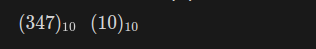### 2. Binary —> 0 & 1 Base: 2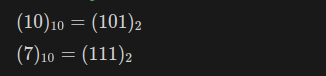Binary is a number system based on the power of 2. It uses only two symbols (0 and 1) to represent numbers. Each digit's value depends on its position, with the rightmost digit representing ones, the next representing twos, and so on.

### 3. Octal —> 0,1,2....7 Base: 8

Octal is a number system based on the power of 8. It uses eight symbols (0-7) to represent numbers. Each digit's value depends on its position, with the rightmost digit representing ones, the next representing eights, and so on.

``````0,1,2,3,4,5,6,7,10,11,12,13,14,15,16,17,20
``````

we do not count numbers which contain 8 and 9

Hexadecimal is a number system based on the power of 16. It uses sixteen symbols (0-9 and A-F) to represent numbers. Each digit's value depends on its position, with the rightmost digit representing ones, the next representing sixteens, and so on.

``````0,1,2,3,4,5,6,7,8,9,A,B,C,D,E,F\$
``````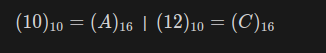## Conversions

### 1. Decimal to base b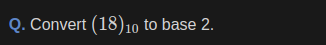The process of converting a decimal number to base-b involves representing the decimal number using digits from 0 to b-1. It includes dividing the decimal number by b, noting the remainders, and concatenating them in reverse order to form the base-b representation.

Keep dividing by base, take remainders and write in reverse order.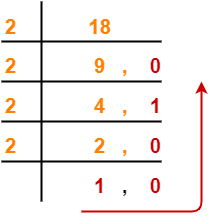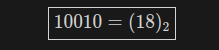### 2. Base to decimal

The process of converting a decimal number to base-b involves representing the decimal number using digits from 0 to b-1. It includes dividing the decimal number by b, noting the remainders, and concatenating them in reverse order to form the base-b representation.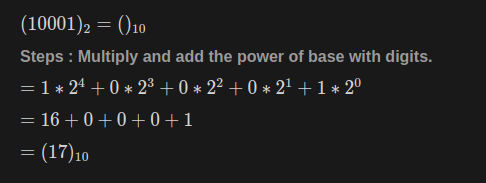Continuing the operator..

### 5. Left Shift operator ( << )

The left shift operator (<<) is a bitwise operator that shifts the bits of a number to the left by a specified number of positions. It effectively multiplies the number by 2 raised to the power of the shift amount. The leftmost bits are discarded, and zeros are filled in from the right. This operation is equivalent to multiplying the number by 2 for each shift.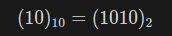now using << 1010 = 10100 | 10100 in decimal is 20
When left shift operator is uded the nubmer is doubled

``````a <<1 = 2a
``````
``````a << b = a*2^b
``````

## 6. Right shift operator ( >> )

The right shift operator (>>) is a bitwise operator that shifts the bits of a number to the right by a specified number of positions. It effectively divides the number by 2 raised to the power of the shift amount. The rightmost bits are discarded, and depending on the sign of the number, either zeros or ones are filled in from the left. This operation is equivalent to dividing the number by 2 for each shift.
Q: 0011001 >> 1 => 001100 => 1100
Two left zeros are ignored in binary numbers.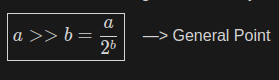### Odd and Even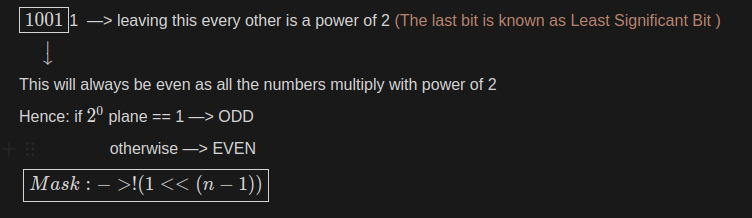10011 --> Leaving this every other is a power of 2 (the last bit is know as Least Significant Bit)
rest of the left bits will always be even as all the numbers multiply with power of 2
Henc if 2 to the powert 0 plane == 1 that will ODD
ohterwise it will be EVEN

``````Mask: -> !(1<<(n-1))
``````

### How to convert Positive number to Negative Number

Step 1: Take the complement of the positive number. In binary representation, this involves flipping all the bits (0s become 1s and 1s become 0s).

Step 2: Add 1 to the complement obtained in Step 1.

The result of this process is the negative representation of the original positive number. This method utilizes the two's complement representation, which is commonly used in computer systems to represent negative numbers. By applying these steps, you effectively invert the sign of the number while maintaining the integrity of the binary representation.

``````EX. (10)_10 = (00001010)_2
Step - 1; 11110101
Step - 2: 1110101 + 1 = (11110110)_2 = (-10)
``````

These steps also known as 2’s complement method.

## Some Shortcut formulas.

### Range Formula ( for n bits )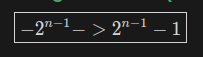### Number of digits in base b of number n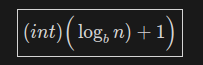### Sum of each row in Pascal’s Triangle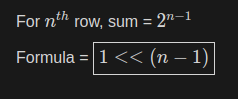### Power of 0

IF n & (n - 1) = 0 // It is power of 2.
In power of 2 number all the numbers are 0 except the most significant bit
The expression "n & (n - 1) = 0" is used to determine if a number is a power of 2. When this expression evaluates to zero, it indicates that the number is a power of 2. In a power of 2 number, all the bits are 0 except for the most significant bit, which is set to 1. By subtracting 1 from a power of 2 number, all the bits to the right of the most significant bit become 1. Therefore, when performing a bitwise AND operation between the number and its decrement, the result will be 0 only if the number is a power of 2.

Ex. 10000000
if we & n with 1111111 ( n - 1 ) it gives 0

``````IF                            0 —> a
a % 4 = 0                     a
a % 4 = 1                     1
a % 4 = 2                     a + 1
a % 4 = 3                     0
``````#### Manish Kumar

I am first year student at KIIT. I am currently learning Web Development, started writing blogs as to record my learning journey, it gives me deeper understanding when I learn and write it later.